# Seven-Year Wilkinson Microwave Anisotropy Probe (WMAP) Observations: Cosmological Interpretation

## Komatsu, E., et.al., 2011, ApJS, 192, 18K

### reprint (2.1 MB) / preprint (1.6 MB) / bundled figures (893 KB) / individual figures / ADS / astro-ph

 WMAP Seven-year Paper Figures, E. Komatsu, et al. Individual figures are provided for use in talks. Proper display of PNG transparency in PowerPoint requires saving files to your computer before Inserting them. Please acknowledge the WMAP Science Team when using these images. Image Credit: NASA / WMAP Science Team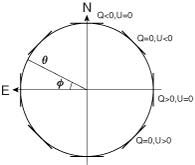Fig.1Coordinate system for Stokes Q and U. We use Galactic coordinates with north up and east left. In this example, Qr is always negative, and Ur is always zero. When Qr > 0 and Ur = 0, the polarization pattern is radial. PNG (17 kb 1824x1542 pixels) PNG (42 kb 3659x3083 pixels) EPS (14 kb)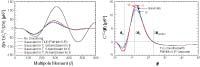Fig.2Temperature-polarization cross correlation with various smoothing functions. (Left) The TE power spectrum with no smoothing is shown in the black solid line. For the other curves, the temperature is always smoothed with a 0.5° (FWHM) Gaussian, whereas the polarization is smoothed with either the same Gaussian (black dashed), Q-band beam (blue solid), V-band beam (purple solid), or W-band beam (red dashed). (Right) The corresponding spatial temperature-Qr correlation functions. The vertical dotted lines indicate (from left to right): the acoustic scale, 2×the acoustic scale, and 2×the horizon size, all evaluated at the decoupling epoch. PNG (60 kb 4145x1389 pixels) PNG (171 kb 8048x2683 pixels) EPS (101 kb)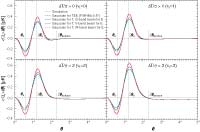Fig.3Predicted temperature peak-polarization cross correlation, as measured by the stacked profile of the transformed Stokes Qr, computed from equation (11) for various values of the threshold peak heights. The temperature is always smoothed with a 0.5° (FWHM) Gaussian, whereas the polarization is smoothed with either the same Gaussian (black dashed), Q-band beam (blue solid), V-band beam (purple solid), or W-band beam (red dashed). (Top left) All temperature hot spots are stacked. (Top right) Spots greater than 1σ are stacked. (Bottom left) Spots greater than 2σ are stacked. (Bottom right) Spots greater than 3σ are stacked. The light gray lines show the average of the measurements from noiseless simulations with a Gaussian smoothing of 0.5° FWHM. The agreement is excellent. PNG (90 kb 3771x2486 pixels) PNG (242 kb 7542x4974 pixels) EPS (135 kb)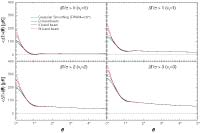Fig.4Predicted temperature peak-temperature correlation, as measured by the stacked temperature profile, computed from equation (13) for various values of the threshold peak heights. The choices of the smoothing functions and the threshold peak heights are the same as in Figure 3. PNG (53 kb 3795x2527 pixels) PNG (151 kb 7500x4995 pixels) EPS (84 kb)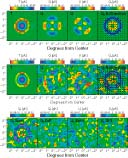Fig.5Stacked images of temperature and polarization data around temperature cold spots. Each panel shows a 5° × 5° region with north up and east left. Both the temperature and polarization data have been smoothed to a common resolution of 0.5°. (Top) Simulated images with no instrumental noise. From left to right: the stacked temperature, Stokes Q, Stokes U, and transformed Stokes Qr (see equation (1)) overlaid with the polarization directions. (Middle) WMAP 7-year V+W data. In the observed map of Qr, the compression phase at 1.2° and the reversal phase at 0.6° are clearly visible. (Bottom) Null tests. From left to right: the stacked Qr from the sum map and from the difference map (V-W)/2, the stacked Ur from the sum map and from the difference map. The latter three maps are all consistent with noise. Note that Ur, which probes the TB correlation (see equation (12)), is expected to vanish in a parity-conserving universe. PNG (69 kb 1664x2048 pixels) PNG (151 kb 3327x4096 pixels) EPS (684 kb)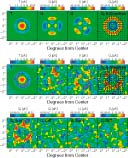Fig.6Same as Figure 5 but for temperature hot spots. PNG (69 kb 1664x2048 pixels) PNG (153 kb 3327x4096 pixels) EPS (594 kb)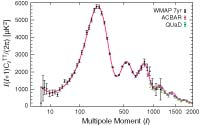Fig.7The WMAP 7-year temperature power spectrum (Larson et al. 2010), along with the temperature power spectra from the ACBAR (Reichardt et al. 2009) and QUaD (Brown et al. 2009) experiments. We show the ACBAR and QUaD data only at l ≥ 690, where the errors in the WMAP power spectrum are dominated by noise. We do not use the power spectrum at l > 2000 because of a potential contribution from the SZ effect and point sources. The solid line shows the best-fitting 6-parameter flat ΛCDM model to the WMAP data alone (see the 3rd column of Table 1 for the maximum likelihood parameters). PNG (25 kb 2064x1292 pixels) PNG (61 kb 4129x2585 pixels) EPS (43 kb)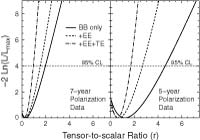Fig.8Limits on the tensor-to-scalar ratio, r, from the polarization data (BB, EE and TE) alone. All the other cosmological parameters, including the optical depth, are fixed at the 5-year best-fit ΛCDM model (Dunkley et al. 2009). The vertical axis shows -2 ln(L/Lmax), where L is the likelihood and Lmax is the maximum value. This quantity may be interpreted as the standard Χ2, as the likelihood is approximately a Gaussian near the maximum; thus, -2 ln(L/Lmax) = 4 corresponds to the 95.4% CL limit. The solid, dashed and dot-dashed lines show the likelihood as a function of r from the BB-only, BB+EE, and BB+EE+TE data. (Left) The 7-year polarization data. We find r < 2.1, 1.6, and 0.93 (95.4% CL) from the BB-only, BB+EE, and BB+EE+TE data, respectively. (Right) The 5-year polarization data. We find r < 4.7, 2.7, and 1.6 (95.4% CL) from the BB-only, BB+EE, and BB+EE+TE data, respectively. PNG (28 kb 2048x1430 pixels) PNG (74 kb 4096x2861 pixels) EPS (41 kb)Fig.9Constraint on the effective number of neutrino species, Neff. (Left) Joint two-dimensional marginalized distribution (68% and 95% CL), showing how a better determination of H0 improves a limit on Ωmh2. (Middle) A correlation between Neff and Ωmh2. The dashed line shows the line of correlation given by equation (58). A better determination of H0 improves a limit on Ωmh2 which, in turn, improves a limit on Neff. (Right) One-dimensional marginalized distribution of Neff from WMAP-only and WMAP+BAO+H0. The 68% interval from WMAP+BAO+H0, Neff = 4.34+0.86-0.88, is consistent with the standard value, 3.04, which is shown by the vertical line. PNG (21 kb 2052x625 pixels) PNG (47 kb 4096x1247 pixels) EPS (49 kb)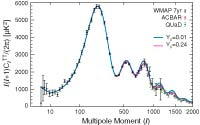Fig.10Primordial helium abundance and the temperature power spectrum. The data points are the same as those in Figure 7. The lower (pink) solid line (which is the same as the solid line in Figure 7) shows the power spectrum with the nominal helium abundance, Yp = 0.24, while the upper (blue) solid line shows that with a tiny helium abundance, Yp = 0.01. The larger the helium abundance is, the smaller the number density of electrons during recombination becomes, which enhances the Silk damping of the power spectrum on small angular scales, l ≥500. PNG (29 kb 2048x1282 pixels) PNG (70 kb 4096x2564 pixels) EPS (47 kb)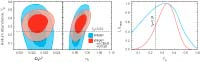Fig.11Constraint on the primordial helium abundance, Yp. (Left) Joint two-dimensional marginalized distribution (68% and 95% CL), showing that Yp and Ωbh2 are essentially uncorrelated. (Middle) A slight correlation exists between Yp and ns: an enhanced Silk damping produced by a larger Yp can be partially canceled by a larger ns. (Right) One-dimensional marginalized distribution of Yp from WMAP-only and WMAP+ACBAR+QUaD. The 68% interval from WMAP+ACBAR+QUaD, Yp = 0.326 ± 0.075 is consistent with the nominal value, 0.24, which is shown by the vertical line. PNG (25 kb 2048x639 pixels) PNG (52 kb 4096x1279 pixels) EPS (32 kb)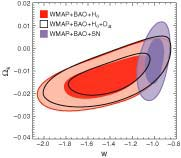Fig.12Joint two-dimensional marginalized constraint on the time-independent (constant) dark energy equation of state, w, and the curvature parameter, Ωk. The contours show the 68% and 95% CL from WMAP+BAO+H0 (red), WMAP+BAO+H0+DΔt (black), and WMAP+BAO+SN (purple). PNG (31 kb 2048x1791 pixels) PNG (75 kb 4096x3582 pixels) EPS (36 kb)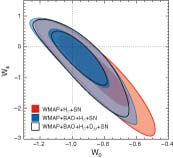Fig.13Joint two-dimensional marginalized constraint on the linear evolution model of dark energy equation of state, w(a) = w0 + wa(1 - a). The contours show the 68% and 95% CL from WMAP+H0+SN (red), WMAP+BAO+H0+SN (blue), and WMAP+BAO+H0+DΔt+SN (black), for a flat universe. PNG (32 kb 2048x1866 pixels) PNG (78 kb 4096x3731 pixels) EPS (29 kb)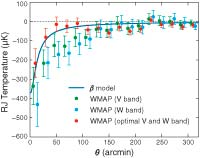Fig.14Angular radial profile of the SZ effect toward the Coma cluster, in units of the Rayleigh-Jeans (RJ) temperature (µK). While the V- (green) and W-band (blue) measurements are contaminated by the CMB fluctuations around Coma, our optimal estimator can separate the SZ effect and CMB when the V- and W-band measurements are combined (red). The solid line shows the best-fitting spherical β model with the core radius of θc = 10.5 arcmin and β = 0.75. The best-fitting central temperature decrement (fit to a β model) is TSZ,RJ(0) = -377±105 µK. Note that 10 arcmin corresponds to the physical distance of 0.195 h-1 Mpc at the location of Coma. The radius within which the mean overdensity is 500 times the critical density of the universe, r500, corresponds to about 50 arcmin. PNG (36 kb 2048x1621 pixels) PNG (75 kb 4096x3241 pixels) EPS (59 kb)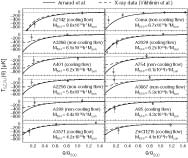Fig.15Angular radial profiles of the SZ effect toward nearby massive clusters (with M500 ≥ 4×1014 h-1 MSUN and z ≤ 0.09), in units of the Rayleigh-Jeans (RJ) temperature (μK). The V- and W-band data are combined optimally to separate the CMB and the SZ effect. All of these clusters have θ500 ≥ 14′, i.e., resolved by the WMAP beam. The masses, M500 are MY given in the 6th column of Table 2 in Vikhlinin et al. (2009a), times hvikhlinin = 0.72 used by them, except for Coma. For Coma, we estimate M500 using the mass-temperature relation given in Vikhlinin et al. (2009a) with the temperature of 8.45 keV (Wik et al. 2009). The dashed lines show the expected SZ effect from the X-ray data on the individual clusters, whereas the solid lines show the prediction from the average pressure profile found by Arnaud et al. (2009). Note that Coma is not included in the sample of Vikhlinin et al. (2009a), and thus the X-ray data are not shown. We find that Arnaud et al.′s profiles overpredict the gas pressure (hence the SZ effect) of non-cooling flow clusters. Note that all cooling-flow clusters are "relaxed," and all non-cooling-flow clusters are "non-relaxed" (i.e., morphologically disturbed), according to the criterion of Vikhlinin et al. (2009a). PNG (53 kb 2046x1724 pixels) PNG (131 kb 4096x3451 pixels) EPS (209 kb)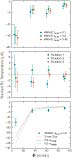Fig.16Average temperature profile of the SZ effect from the stacking analysis, in units of the Rayleigh-Jeans (RJ) temperature (µK), at θ = 7, 35, 63, and 91 arcmin. The V- and W-band data are combined using the optimal estimator. (Top) The SZ effect measured from the locations of clusters of galaxies. The results with three different maximum redshifts, zmax = 0.1 (blue; left), 0.2 (green; middle), and 0.45 (red; right), are shown. The error bars include noise due to the CMB fluctuation, and thus are correlated (see equation (82) for the correlation matrix). (Middle) A null test showing profiles measured from random locations on the sky (for zmax = 0.2; the number of random locations is the same as the number of clusters used in the top panel). Three random realizations are shown. Our method does not produce biased results. (Bottom) The measured profile (zmax = 0.2) is compared with the model profiles derived from X-ray observations (Arnaud et al. 2009) and theoretically calculated from hydrostatic equilibrium (Komatsu & Seljak 2001) with two different concentration parameters. Note that the model profiles are calculated also for zmax = 0.2 but have not been multiplied by the best-fitting normalization factors given in Table 12. PNG (20 kb 939x2048 pixels) PNG (51 kb 1879x4096 pixels) EPS (69 kb)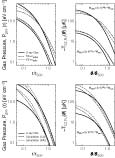Fig.17Gas pressure profiles of clusters of galaxies, Pgas(r), at z = 0.1, and the projected profiles of the SZ effect, ΔTSZ(θ) (Rayleigh-Jeans temperature in µK). (Top Left) The gas pressure profiles. The upper and bottom set of curves show M500 = 3×1014 and 3 × 1013h-1 Msun, respectively. The horizontal axis shows radii scaled by the corresponding r500 = 0.78 and 0.36 h-1 Mpc, respectively. The solid lines show Pgas(r) = Pe(r)/0.518 derived from X-ray observations (Arnaud et al. 2009), while the dotted and dashed lines show Pgas(r) predicted from hydrostatic equilibrium (Komatsu & Seljak 2001) with NFW concentration parameters of Seljak (2000) and Duffy et al. (2008), respectively. (Top Right) The projected SZ profiles computed from the corresponding curves in the top left panel and equation (87). The horizontal axis shows angular radii scaled by θ500 = r500/DA, which is 10 and 4.7 arcmin for M500 = 3 × 1014 and 3 × 1013 h-1 Msun, respectively. (Bottom Left) Same as the top left panel, but the dotted and dashed lines show Pgas(r) predicted from “Cooling+Star Formation” and “Non-radiative” simulation runs by Nagai et al. (2007). (Bottom Right) Same as the top right panel, but the dotted and dashed lines are computed from the corresponding curves in the bottom left panel and equation (87). PNG (47 kb 1485x2048 pixels) PNG (110 kb 2970x4096 pixels) EPS (98 kb)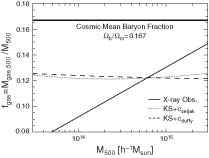Fig.18Gas mass fraction as a function of M500. The thick horizontal line shows the cosmic mean baryon fraction, Ωb/Ωm = 0.167. The solid line shows the gas mass fraction, fgas = Mgas,500/M500, derived from X-ray observations (Vikhlinin et al. 2009a), while the dotted and dashed lines show fgas predicted from hydrostatic equilibrium (Komatsu & Seljak 2001) with NFW concentration parameters of Seljak (2000) and Duffy et al. (2008), respectively. PNG (22 kb 2048x1548 pixels) PNG (56 kb 4096x3097 pixels) EPS (39 kb)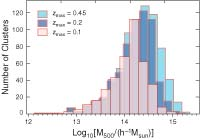Fig.19Distribution of M500 estimated from clusters in the catalog using the measured X-ray luminosities in 0.1-2.4 keV band, LX, and equations (89) and (C2). The light blue, dark blue, and pink histograms show zmax = 0.45, 0.2, and 0.1, respectively. PNG (34 kb 2048x1433 pixels) PNG (79 kb 4096x2866 pixels) EPS (46 kb)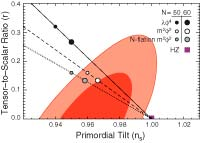Fig.20Two-dimensional joint marginalized constraint (68% and 95% CL) on the primordial tilt, ns, and the tensor-to-scalar ratio, r, derived from the data combination of WMAP+BAO+H0. The symbols show the predictions from “chaotic” inflation models whose potential is given by V (Φ) ∝ Φα (Linde 1983), with α = 4 (solid) and α = 2 (dashed) for single-field models, and α = 2 for multi-axion field models with β = 1/2 (dotted; Easther & McAllister 2006). PNG (29 kb 2048x1463 pixels) PNG (70 kb 4096x2926 pixels) EPS (38 kb)
A service of the HEASARC and of the Astrophysics Science Division at NASA/GSFCHEASARC Director: Dr. Alan P. Smale
LAMBDA Director: Dr. Eric R. Switzer
NASA Official: Dr. Eric R. Switzer
Web Curator: Mr. Michael R. Greason